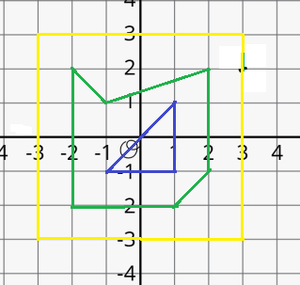# Find number of Polygons lying inside each given Polygons on Cartesian Plane

• Last Updated : 14 Jun, 2022

Given N non-intersecting nested polygons, and a 2D array arr[][] of pairs, where each cell of the array represents the coordinates of the vertices of a polygon. The task is to find the count of polygons lying inside each polygon.

Examples:

Input: N = 3, arr[][][] = {{{-2, 2}, {-1, 1}, {2, 2}, {2, -1}, {1, -2}, {-2, -2}}, {{-1, -1}, {1, -1}, {1, 1}}, {{3, 3}, {-3, 3}, {-3, -3}, {3, -3}}}
Output: 1 0 2
Explanation:1. The polygon represented at index 0, is the green-colored polygon shown in the picture above.
2. The polygon represented at index 1, is the blue-colored polygon shown in the picture above.
3. The polygon represented at index 2, is the yellow-colored polygon shown in the picture above.

Therefore, there is 1 polygon inside the polygon at index 0, and 0 polygons inside the polygon at index 1, and 2 polygons inside the polygon at index 2.

Approach: The above problem can be solved based on the following observations:

1. It can be observed that the polygon lying outside will have the largest X- coordinates. The maximum X-coordinates of the polygon will decrease, as one will go towards the inside, as it is given that polygons are nested and non-intersecting.
2. Therefore, sorting the polygons by the maximum X-coordinate will give the correct order of the polygons lying.

Follow the steps below to solve the problem:

• Initialize a 2D array say maximumX[][] of size N*2 to store the pair of maximum X-coordinates of a polygon and the index value of the polygon.
• Iterate over the array arr[][] using the variable, i perform the following steps:
• Initialize a variable, say maxX, to store the maximum X-coordinate of the current polygon.
• Iterate over the array arr[i] using the variable j and in each iteration update the maxX as maxX = max(maxX, arr[i][j]).
• Assign the pair {maxX, i} to maximumX[i].
• Sort the array of pairs in descending order by the first element using a custom comparator.
• Initialize an array, say res[], to store the count of polygons lying inside the current polygon.
• Iterate over the range [0, N-1] using the variable i and in each iteration assign N-i-1 to res[maximumX[i]], as there are N-i-1 polygons lying inside the maximumX[i]th polygon.
• Finally, after completing the above steps, print the array res[] as the answer.

Below is the implementation of the above approach:

## C++

 `// C++ program for above approach``#include``using` `namespace` `std;` `// Function to calculate maximum sum``void` `findAllPolygons(``int` `N,``                     ``vector > > arr)``{``  ` `    ``// Stores the maximum X co-``    ``// ordinate of every polygon``    ``vector > maximumX(N);` `    ``// Traverse the array arr[][]``    ``for` `(``int` `i = 0; i < N; i++) {` `        ``// Stores the max X co-``        ``// ordinate of current``        ``// polygon``        ``int` `maxX = INT_MIN;` `        ``// Traverse over the vertices``        ``// of the current polygon` `        ``for` `(``int` `j = 0; j < arr[i].size(); j++) {``            ``// Update maxX``            ``maxX = max(maxX, arr[i][j]);``        ``}` `        ``// Assign maxX to maximumX[i]``        ``maximumX[i].first = maxX;` `        ``// Assign i to maximumX[i]``        ``maximumX[i].second = i;``    ``}` `    ``// Sort the array of pairs``    ``// maximumX in descending``    ``// order by first element``    ``sort(maximumX.rbegin(), maximumX.rend());` `    ``// Stores the count of``    ``// polygons lying inside``    ``// a polygon``    ``vector<``int``> res(N, 0);` `    ``// Traverse the maximumX array``    ``for` `(``int` `i = 0; i < N; i++) {``        ``// Update value at``        ``// maximumX[i] in``        ``// res``        ``res[maximumX[i].second] = N - 1 - i;``    ``}` `    ``// Print the array array res``    ``for` `(``int` `i = 0; i < N; i++) {``        ``cout << res[i] << ``" "``;``        ``// System.out.print(res[i] + " ");``    ``}``}` `// Driver Code``int` `main()``{``    ``int` `N = 3;``    ``vector > > arr = {``        ``{ { -2, 2 },``          ``{ -1, 1 },``          ``{ 2, 2 },``          ``{ 2, -1 },``          ``{ 1, -2 },``          ``{ -2, -2 } },``        ``{ { -1, -1 }, { 1, -1 }, { 1, 1 } },``        ``{ { 3, 3 }, { -3, 3 }, { -3, -3 }, { 3, -3 } }``    ``};` `    ``findAllPolygons(N, arr);``    ``return` `0;``}` `// The code is contributed by Gautam goel (gautamgoel962)`

## Java

 `// Java program for above approach``import` `java.util.*;` `class` `GFG {` `    ``// Function to sort a 2D``    ``// array using custom a comparator``    ``public` `static` `void` `sort2d(``int``[][] arr)``    ``{``        ``Arrays.sort(arr, ``new` `Comparator<``int``[]>() {``            ``public` `int` `compare(``final` `int``[] a,``                               ``final` `int``[] b)``            ``{``                ``if` `(a[``0``] < b[``0``])``                    ``return` `1``;``                ``else``                    ``return` `-``1``;``            ``}``        ``});``    ``}` `    ``// Function to calculate maximum sum``    ``static` `void` `findAllPolygons(``int` `N,``                                ``int``[][][] arr)``    ``{``        ``// Stores the maximum X co-``        ``// ordinate of every polygon``        ``int``[][] maximumX = ``new` `int``[N][``2``];` `        ``// Traverse the array arr[][]``        ``for` `(``int` `i = ``0``; i < N; i++) {` `            ``// Stores the max X co-``            ``// ordinate of current``            ``// polygon``            ``int` `maxX = Integer.MIN_VALUE;` `            ``// Traverse over the vertices``            ``// of the current polygon` `            ``for` `(``int` `j = ``0``; j < arr[i].length; j++) {``                ``// Update maxX``                ``maxX = Math.max(maxX, arr[i][j][``0``]);``            ``}` `            ``// Assign maxX to maximumX[i]``            ``maximumX[i][``0``] = maxX;` `            ``// Assign i to maximumX[i]``            ``maximumX[i][``1``] = i;``        ``}` `        ``// Sort the array of pairs``        ``// maximumX in descending``        ``// order by first element``        ``sort2d(maximumX);` `        ``// Stores the count of``        ``// polygons lying inside``        ``// a polygon``        ``int``[] res = ``new` `int``[N];` `        ``// Traverse the maximumX array``        ``for` `(``int` `i = ``0``; i < N; i++) {``            ``// Update value at``            ``// maximumX[i] in``            ``// res``            ``res[maximumX[i][``1``]] = N - ``1` `- i;``        ``}` `        ``// Print the array array res``        ``for` `(``int` `i = ``0``; i < N; i++) {``            ``System.out.print(res[i] + ``" "``);``        ``}``    ``}` `    ``// Driver Code``    ``public` `static` `void` `main(String[] args)``    ``{` `        ``int` `N = ``3``;``        ``int``[][][] arr = {``            ``{ { -``2``, ``2` `}, { -``1``, ``1` `}, { ``2``, ``2` `}, { ``2``, -``1` `}, { ``1``, -``2` `}, { -``2``, -``2` `} },``            ``{ { -``1``, -``1` `}, { ``1``, -``1` `}, { ``1``, ``1` `} },``            ``{ { ``3``, ``3` `}, { -``3``, ``3` `}, { -``3``, -``3` `}, { ``3``, -``3` `} }``        ``};` `        ``findAllPolygons(N, arr);``    ``}``}`

Output

`1 0 2 `

Time Complexity: O(M + N log N), Where M maximum size of a polygon
Auxiliary Space: O(N)

My Personal Notes arrow_drop_up